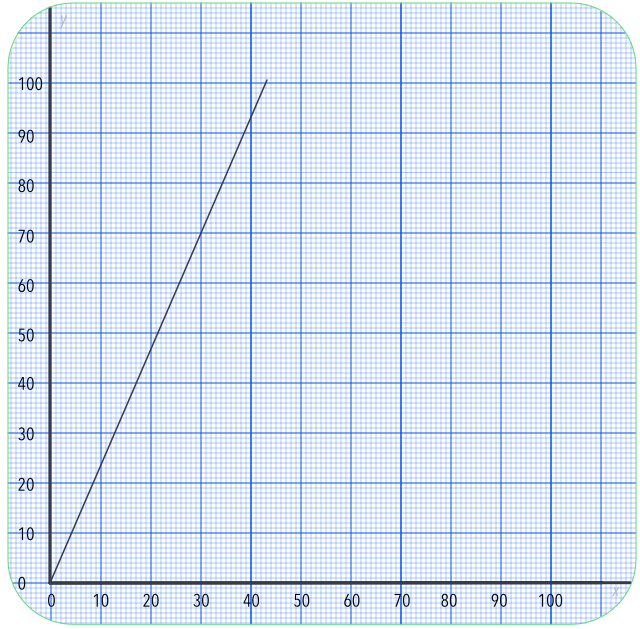Relating Ratios and Functions

# Relating Ratios and Functions

GCSE(F),

A ratio can be turned into a function. To turn a ratio into a function, one side of the ratio must be set to 1. A ratio of 3 : 7 can be turned into a ratio of 1 : frac(7)(3).

With one side of the ratio set to 1, it can now be mapped as x -> frac(7)(3)x, or described as f(x) = frac(7)(3)x. The function can be drawn as a graph to show, for example, conversion rates between units (miles and kilometres) or currencies.The gradient of the graph gives the ratio. The graph will also pass through (0,0).

## Examples

1. A ratio 1 : 5 is mapped as a function, and plotted onto a graph. What is the gradient of the graph?.

The function will be plotted as x -> 5x. The gradient is given by frac(text(up))(text(along)) = frac(5)(1).